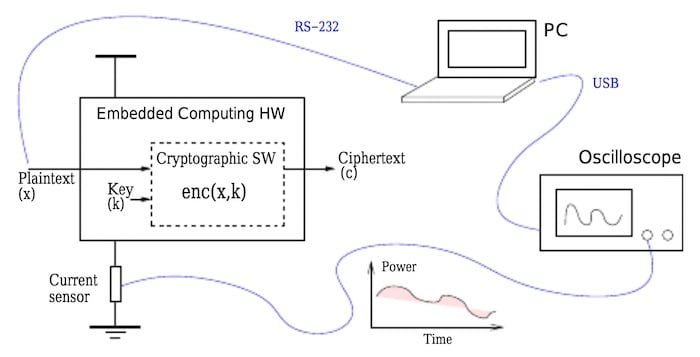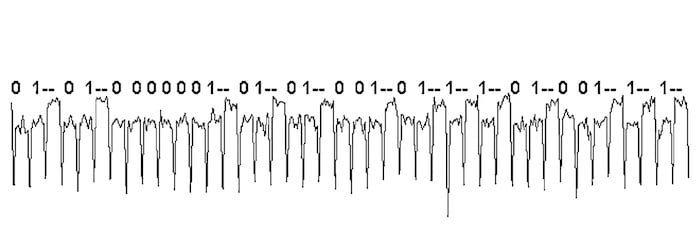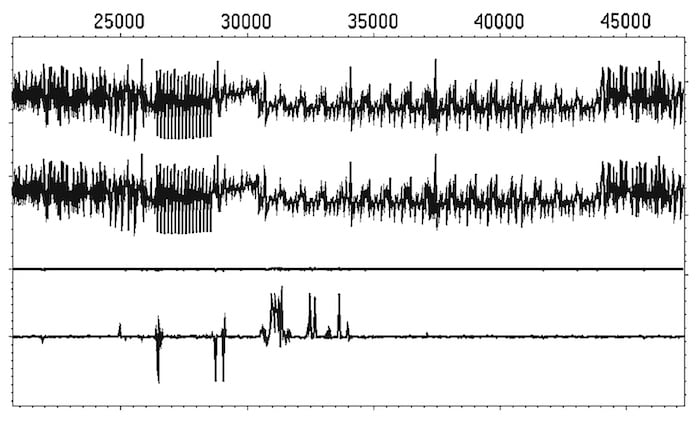Technical Article

# An Introduction to Power Analysis Side Channel Attacks

March 26, 2023 by Jake Hertz

## Learn the basics of how power-based side-channel attacks work and the different types of power analysis attacks, including simple power analysis (SPA), differential power analysis (DPA), and correlational power analysis (CPA).

Previously, I provided an introduction to the field of side-channel attacks as a whole, including why they can pose a significant security threat at the hardware level.

While there are many different forms of side-channel attacks, including electromagnetic (EM), acoustic, and timing, however, one of the most common and stronger types of attacks is power-based side-channel attacks.

In this article, we’ll talk about how a power attack works and look at specific forms of power attacks that have been used to break cryptography in the past.

### Concept Behind Power Side Channel Attacks

The entire concept of power-based side-channel attacks, which a typical setup can be seen in Figure 1, is built on the assumption that, in complementary metal-oxide-semiconductor (CMOS) devices, there is a correlation between the level of power consumption and the operations that the device is performing.##### Figure 1. Test setup for a power-based side-channel attack. Image used courtesy of Wang et al

That is to say, the power consumed by a circuit will vary according to the activity of the individual transistors.

With this understanding, attackers have come to find that the power consumption of an IC is a side channel. Basically, it contains leakage information about what is happening inside of the IC, what data is being processed, and what operations are being performed.

Most power analysis attacks work by having an attacker monitor the device’s power rails during operation (most commonly with an oscilloscope), looking for either current draw or fluctuations in voltage. The attacker will then take its data and either run a series of statistical analyses to correlate their findings to information about the device’s cryptographic operation.

To this end, there are three major forms of power-based attacks worth discussing:

• Simple power analysis (SPA)
• Differential power analysis (DPA)
• Correlational power analysis (CPA)

### Simple Power Analysis (SPA) Attack

The most rudimentary type of power attack is simple power analysis (SPA)

SPA attacks are a method of power-based side-channel attacks that involve interpreting a device’s power consumption measurements directly and mostly visually. These kinds of attacks often require prior knowledge of the kind of cryptographic algorithm that a device is running in order to correlate the measured power consumption to actual mathematical operations.

One famous example of SPA breaking a cryptographic algorithm is in smartcards running RSA (Rivest–Shamir–Adleman). RSA is a public key cryptographic algorithm that operates by performing a series of modular exponentiation loops on the cryptographic key, each consisting of a number of multiplication and squaring operations. It is known that a square operation is performed in every iteration of the exponentiation loop, while multiplications are only performed when a bit of the exponent is 1.

Figure 2 shows a segment of a power trace in a modular exponentiation loop of RSA (i.e, a series of squares and multiplications).##### Figure 2. SPA leaks from an RSA implementation. Image used courtesy of Kocher et al

As can be seen from the power trace, the multiplication operations consume more power than the squaring operations, which results in a taller spike in the power trace. From this trace, along with our knowledge of the RSA algorithm, we can then simply determine that each spike in power (i.e. a multiplication operation) correlates to a ‘1’ bit in the secret key exponent. A ‘0’, in this case, appears as a shorter bump without a subsequent taller one. In this way, SPA is able to recover a secret cryptographic key from RSA.

### Differential Power Analysis (DPA) Attacks

SPA works best on very simple electronic systems like smart cards, as the lack of functionality allows one to directly and visually correlate power traces to specific operations. In more realistic and complex systems, many operations are occurring in parallel, all drawing from the same power rail - making it virtually impossible to decipher anything visually.

For more complex systems, one of the most powerful power attacks is differential power analysis (DPA)

DPA is a type of power-based side-channel attack that uses statistical methods for analyzing sets of measurements to identify data-dependent correlations (Figure 3).##### Figure 3. The results of a DPA test. From top to bottom: the output of the first AES S-box when the LSB is 1, the same output when the LSB is 0, the difference between the outputs, and the difference magnified 15x. Image used courtesy of Kocher et al

The method largely consists of collecting traces, like in SPA, splitting them into individual subsets as defined by a selection function, and computing the difference in their averages. If there is no correlation between the subsets, the difference will be zero - if there is a correlation between sets, the difference will be some non-zero number. Over a large enough dataset, these correlations become discernible regardless of the amount of noise in the data.

This knowledge can be extrapolated to uncover cryptographic keys in algorithms like the AES (advanced encryption standard) and DES (data encryption standard). In the case of AES with a 128-bit key, trying to guess the key by brute force would take 2^128 attempts which would be computationally infeasible. Using DPA, however, it has been shown that the key can be deciphered in just 16*256 attempts, which is trivially easy.

Further discussion into the specific details of how DPA breaks AES or DES is outside of the scope of this article, so for more information, I suggest reading the work of Kocher et al here

### Correlation Power Analysis (CPA) Attacks

Another powerful type of power attack, which is actually a variation of DPA, is correlation power analysis (CPA)

CPA works by trying to create a power model of the device so that the attacker can find a statistical correlation between the predicted output and the power consumption of the device. The more accurate the power model, the stronger the correlation will be.

One type of power model that gets widely used in CPA is the hamming weight model, which makes the assumption that the number of ‘1’ bits in the cryptographic key will directly correlate to the device’s power consumption during encryption. Using this model, the attacker will provide different keys to the device, and the Hamming weights are compared to voltage levels of power traces by finding the correlation between the modeled power unit values and the actual power consumed.

If a correlation is found, then an attacker works to gather a large number of traces resulting from different predicted secret key inputs and the correct key will result in the highest level of statistical correlation.

For more detailed information on CPA attacks, I recommend reading Power Analysis Attacks by Mangard et al.

All in all, power-based side-channel attacks are amongst the most powerful forms of hardware-level attack known so far. Attacks like DPA and CPA have been proven to break encryption algorithms that were previously thought to be unbreakable, such as AES and DES.